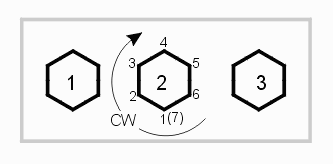## Sample Polygon Data Structure

Consider the filled region shown below at left. It can be represented as a Leonov polygon: a rectangular parent polygon containing three child polygons. This is a common occurrence in Gerber files with negative ground planes.The data returned by GBRUnion DLL is organized as follows:

```
typedef struct
{
int    Layer;             -- if Leonov needs layer for embedding level output
int    Vsize;             -- 5 if circular; 2 if no arcs in polygon
int    VCnt;              -- Vertex count of single or container polygon
double *XY;               -- list of XY coordinates for the container (parent) polygon
int    HoleCnt;           -- Number of holes in container polygon
int    *HoleVCnts;        -- list of vertex counts for each contained hole
double **HoleXYs;         -- list of XY data for each contained hole
} ACS_GBRUNION_Polygon;

```

Layer - for Leonov polygons a layer is needed to hold the embedded polygons.

Vsize - either 2 or 5 depending on whether the polygon has only straight edges (2) or circular data (5).

Vcnt - the number of vertices in the parent. Note that since we adhere to the GDSII description of polygons for our internal database the first vertex is counted twice - once at the beginnning and once at the end. In this example Vcnt=5double *XY - coordinates of the parent polygon.

holecount - the number of child polygons. Note that child polygons may not touch the parent nor touch each other. In this example holecnt = 3

*HoleVCnts - a list of the vertex counts for the child polygons. In this example we have three children and each has 7 vertcies. In this example the list would look like: 7 7 7**HoleXYs - list of the XY coordinates for the children polygons.

If a polygon is of type re-entrant or butting, then it won't have any internal holes and only Vsize, VCnt and *XY are of interest.